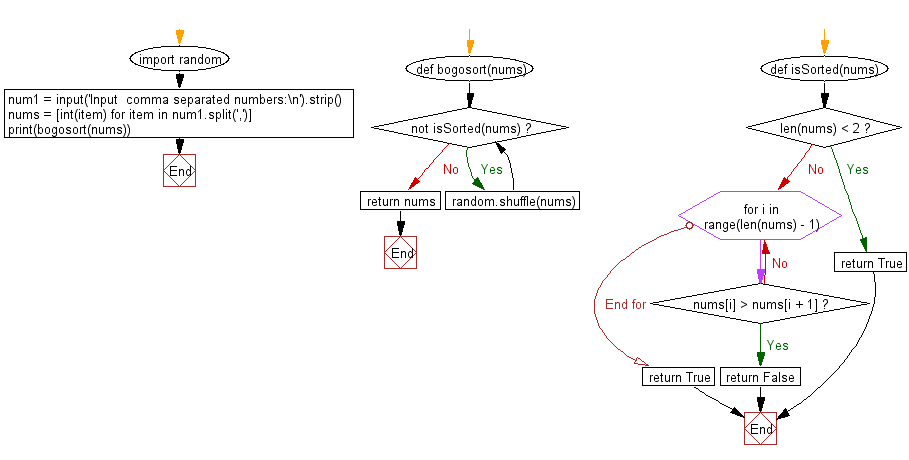﻿ Python: Sort a list of elements using Bogosort sort - w3resource# Python: Sort a list of elements using Bogosort sort

## Python Search and Sorting : Exercise-12 with Solution

Write a Python program to sort a list of elements using Bogosort sort.
In computer science, Bogosort is a particularly ineffective sorting algorithm based on the generation and test paradigm. The algorithm successively generates permutations of its input until it finds one that is sorted. It is not useful for sorting, but may be used for educational purposes, to contrast it with other more realistic algorithms. Two versions of the algorithm exist: a deterministic version that enumerates all permutations until it hits a sorted one, and a randomized version that randomly permutes its input. An analogy for the working of the latter version is to sort a deck of cards by throwing the deck into the air, picking the cards up at random, and repeating the process until the deck is sorted. Its name comes from the word bogus.

Sample Solution:

Python Code:

``````import random

def bogosort(nums):
def isSorted(nums):
if len(nums) < 2:
return True
for i in range(len(nums) - 1):
if nums[i] > nums[i + 1]:
return False
return True

while not isSorted(nums):
random.shuffle(nums)
return nums
num1 = input('Input  comma separated numbers:\n').strip()
nums = [int(item) for item in num1.split(',')]
print(bogosort(nums))
```
```

Sample Output:

```Input  comma separated numbers:
15, 25, 37, 65, 75, 12, 89
[12, 15, 25, 37, 65, 75, 89]
```

Flowchart:Python Code Editor:

What is the difficulty level of this exercise?

Test your Programming skills with w3resource's quiz.

﻿

## Python: Tips of the Day

Use Reversed() In for Loops:

```>>> tasks = ['laundry', 'picking up kids', 'gardening', 'cooking']